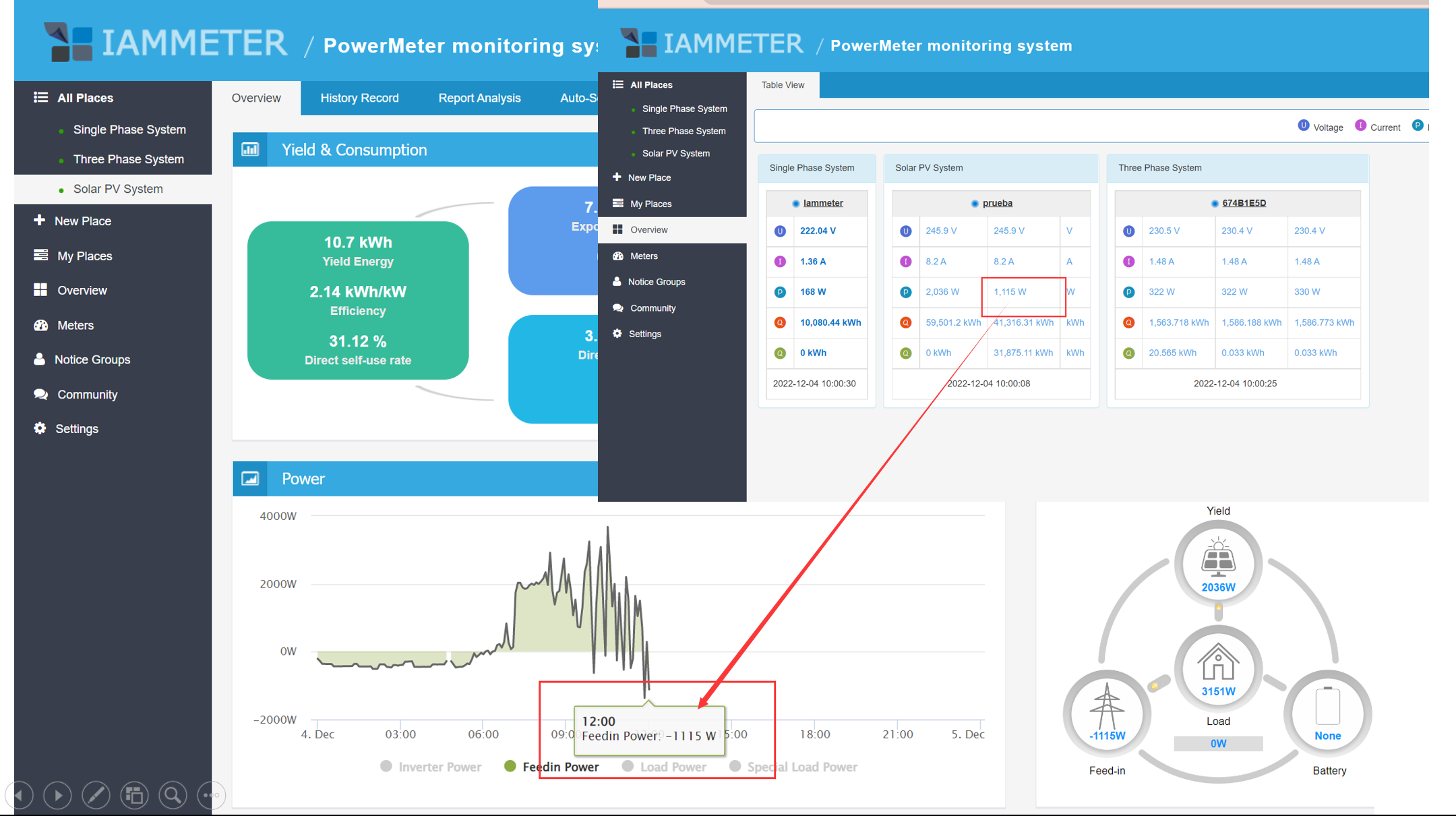### Positive vs negative power/energy

I am using two meters, a Chint/Huawei DTSU666-H and a WEM3080T. While DTSU666-H uses a negative sign for grid consumption power/energy and a positive sign for export power/energy, WEM3080T does it the other way around. CTs are mounted in the correct direction for both. I think WEM3080T does it the right way in accordance with the OBIS codes:

1.8.0/1.8.1/1.8.2 (import): positive active energy (A+)

2.8.0 (export): negative active energy (A-)

Huawei writes in their FusionSolar portal:

"By default, the power is a positive value when electricity is fed in to the grid."

So it looks like it can be changed. But is there actually a convention for positive active power/energy to be export power/energy?

4
387
2
0
0

###### Sort replies by:

Hi:

Sorry,I do not know what is the ""OBIS codes" exactly, I will search it later.

let me introduce how we judge positive and negative power:
power =U*I*cos(phase angle between voltage and current)
In short
It depends on the phase angle difference between the voltage and current.
When the energy meter samples the voltage and current by the ASIC(introduced here ), it will also get the phase angle difference between the voltage and current.

Both voltage and current are sine waves, when there is only a resistor load(no capacitor load and inductance load), there will be no phase angle between current and voltage.
When there is an inductance load or capacitor load in your system, there will be a phase angle difference.
Usually, it would be from -90°-90° and the cos(phase angle difference) is positive, this is the definition of the power factor.

When there is solar PV inverter is installed, it may feed in energy to the grid when the load power is less than the solar yield power.
At this time, the phase angle difference would be [90-270], so the grid power will be calculated as negative.

But the phase angle reference of both voltage and current is determined by the installation.
For example, when you switch the voltage input from UL and UN, the voltage phase angle will also switch 180 degrees, or when you change the CT direction, the phase angle of the current will also switch 180 degrees.

each positive power will be counted into the first kWh register(we call it forward energy)

each positive power will be counted into the second kWh register(we call it reverse energy)

I am using two meters, a Chint/Huawei DTSU666-H and a WEM3080T. While DTSU666-H uses a negative sign for grid consumption power/energy and a positive sign for export power/energy, WEM3080T does it the other way around. CTs are mounted in the correct direction for both. I think WEM3080T does it the right way in accordance with the OBIS codes:1.8.0/1.8.1/1.8.2 (import): positive active energy (A+)2.8.0 (export): negative active energy (A-)Huawei writes in their FusionSolar portal:"By default, the

Check this out for more on OBIS.

In fact, we do not know about OBIS before.
This is my point.
1 OBIS gives a method of classification. In fact, I do not know the physical meaning of the " negative active energy" in the OBIS(such as whether it regards solar PV or not).
2 Most of the solar inverter`s portal will define the exported energy as positive because this meets the requirements of most customer that install the solar PV system.
The power that feeds into the grid is positive.
In fact,we also provide a feedin power display in the overview page.
The feeding power is simply the opposite value of the corresponding grid power.Check this out for more on OBIS.

OBIS is very common in Austria and Germany where I live (I don't know about other countries). Meters in those countries typically show grid consumed energy (positive active energy, A+) as 1.8.0. If you have a two tariff meter, it shows consumed energy during tariff 1 times as 1.8.1 and measured consumed energy during tariff 2 times as 1.8.2.

And if you have a solar system you'll get a bidirectional meter which can also be a 1 or 2 tariff counter (so either showing 1.8.0 or 1.8.1/1.8.2) which additionally shows fed-in energy (negative active energy, A-) as 2.8.0.

Interesting that there indeed seems to be a difference in how positive and negative power/energy is defined for meters and solar systems.

Looks like you are new here. Register for free, learn and contribute.